# Coin Toss Genetics### AUSTRALIAN CURRICULUM ALIGNMENT

• Identify, research and construct questions for investigation; propose hypotheses; and predict possible outcomes (ACSBL061)
• Conduct investigations, including the use of probabilities to predict inheritance patterns, real or virtual gel electrophoresis, and population simulations to predict population changes, safely, competently and methodically for the collection of valid and reliable data (ACSBL063)

### BACKGROUND

Simulating genetics can be done using simple coin procedures. This practical introduces students to the idea of statistical probability in genetics and simulates a monohybrid cross. Students will use the head side of the coin to represent a dominant allele for a trait, and the tail to represent the corresponding recessive allele. To make this practical more engaging, students may even simulate a cross prior to conducting it in the lab. Drosophila vestigial wings make a great model for this exercise. In this case, the heads can represent the allele for normal (wild type) wings and tails can represent the allele for vestigial wings. Wisconsin Fast Plants® crosses are also perfect for this exercise, to explore the probability of purple stem allele.

A coin represents a diploid, heterozygous cell with its heads and tails sides. The act of tossing the coin to land on heads or tails, provides an apt representation of the segregation of alleles during meiosis in the formation of gametes. Tossing two coins and combining the results (HH, HT, or TT) clearly demonstrates the recombination of alleles during fertilization and the establishment of the next generation genotype.

### METHOD- STUDENT ACTIVITY

Procedure 1: Statistical Probability Reasoning

1. If you toss the coin, what are the chances that it will land on heads? Record your predictions and explain your reasoning. Toss a single coin 5X and record the results Table 1.

2. If you toss 2 coins, what are the chances you will get 2 heads? Record your predictions and explain your reasoning. Find a partner for this exercise and toss 2 coins 5X each. Discuss the results with your partner and whether this meets your prediction. Combine your partner’s results with your own and record the results in Table 1. You should have a set of 10 coins toss results.
3. Are the chances of getting 2 tails the same as getting 2 heads, if you toss 2 coins? Record your prediction and explain your reasoning. Toss two coins 5X and record the results in Table 1.
4. What are the chances of getting a head and a tail from tossing 2 coins? Discuss your predictions with the class. Toss 2 coins 5X and record the results in Table 1.
5. Your teacher will take you through the correct answers for the chances of different coin combinations

Procedure 2: Monohybrid Cross Simulation

1. Determine all possible results of tossing two coins. Record the results in Table 2.
2. Using heads to represent the dominant allele (A) and tails to represent the recessive allele (a), the heads and tails of the coins are used to simulate the crossing of a heterozygous F1 to produce an F2: A/a x A/a.
3. Working with your partner, simultaneously toss your coins and record the results in a table. Repeat until you have conducted one hundred tosses.
4. Total your results and record them in Table 3. Record the results of the Class Totals in Table 3.
5. Determine the Total F2 with Dominant Phenotype by combining the total results of the classes of A/A and A/a.
6. The Total F2 with Recessive Phenotype is the Class Total of a/a results.
7. What is the predicted Phenotype Ratio? Actual Phenotype Ratio = Total F2 with Dominant Phenotype/ Total F2 with Recessive Phenotype: Total F2 with Recessive Phenotype/ Total F2 with Recessive Phenotype = ___ : ___

### OBSERVATION AND RESULTS

Procedure 1: Statistical Probability Reasoning

Individual results will vary; however, results will approximately align with the statistical probability.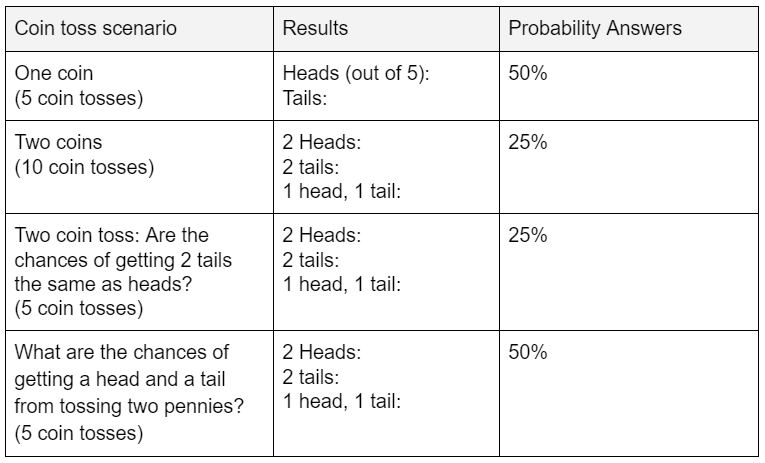Table 1: Statistical Probability Results

Procedure 2: Monohybrid Cross Simulation

2 Coin Toss: Below are the possible results of tossing 2 coins.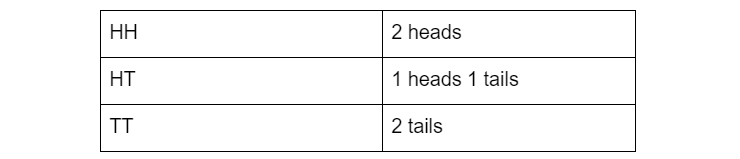Table 2: Coin Toss Results

Cross Monohybrid Simulation Table: Individual results will vary. Students may record their results in the table below.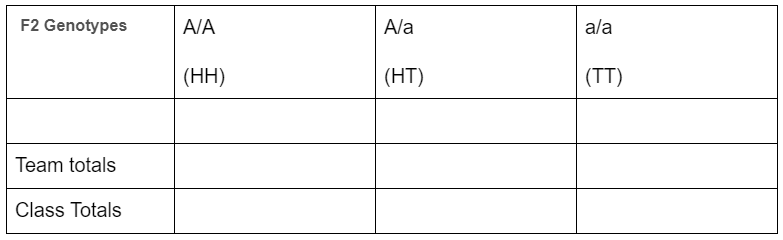Table 3: Cross Simulation

• The total F2 with Dominant Phenotype results will vary depending on the coin toss results.
• The total F2 with Recessive Phenotype results will vary depending on the coin toss results.
• The predicted Phenotype Ratio should be 3:1.
• The Actual Phenotype Ratio results will vary depending on the coin toss results.

### INVESTIGATIONS

• Challenge students to explain the variation of Actual Phenotype Ratio and Predicted Phenotype Ratio. Students should understand their results are influenced by chance.

• Challenge students to suggest potential ideas to make the actual ratio and predicted ratio match more. One potential method to do this it to generate a larger data set.

### EXTENSION EXERCISE

To explore genetic crosses further using coins, you may like to ask students to complete a coin simulation of a dihybrid cross using 2 different types of coins.

1. 45 mins

#####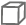Material List
1. 1 Silver Coin (10 or 20 cent)
#####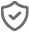Safety Requirements
1. Wear appropriate personal protective equipment (PPE).
2. Ensure that students understand and adhere to safe conduct practices within the classroom.